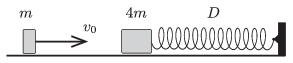Mathematical and Physical Journal
for High Schools
Issued by the MATFUND Foundation
 Already signed up? New to KöMaL?

#Problem P. 4707. (February 2015)

P. 4707. An object of mass $\displaystyle m=0.2$ kg and another one of mass $\displaystyle 4m$ can move frictionlessly along a horizontal surface. The greater mass object is attached to an unstretched spring of spring constant $\displaystyle D=320$ N/m. (The spring is designed for both compression and tension.) The smaller object collides head-on at a speed of $\displaystyle v_0=5$ m/s with the stationary heavy object. The collision is totally elastic.$\displaystyle a)$ What is the maximum compression of the spring?

$\displaystyle b)$ What will the maximum speed of the object attached to the spring be, and what is the greatest extension of the spring?

(4 pont)

Deadline expired on March 10, 2015.

### Statistics:

 97 students sent a solution. 4 points: 51 students. 3 points: 19 students. 2 points: 9 students. 1 point: 17 students. 0 point: 1 student.

Problems in Physics of KöMaL, February 2015